# Writing a formula for the ROI calculator

Once you've added an ROI calculator to your Qwilr Page, you'll need to create a formula for the calculation. If you've never done that before, read on.

For this guide, we're assuming that you've already added the ROI calculator to your page and set up your variables. For details on those steps, please see this guide.

To add or edit a formula, click on the ROI Options icon. Click anywhere in the formula field to bring up the editing toolbar.## Parts of a formula

Your ROI formula can have up to 3 parts:

### 1. Variables

Variables represent the actual numbers in your formula. ( See this guide for more details on setting up variables.)

They look like a letter in two sets of curly brackets, like this: {{A}}. (The calculator will assign a letter to each value automatically.)

You can add a variable to your formula by clicking the Variables icon and then choosing from your list of variables.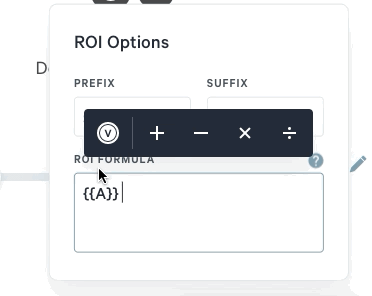Note: The calculator uses these symbols instead of actual numbers to keep the calculation flexible. That way, you and your client can move the slider and adjust the parameters as needed, and see the numbers change.

Note: If you're using one of the preset calculators, we recommend editing the existing variables instead of deleting them to add new ones. Deleting variables will cause the preset formula to break.

### 2. Operators

Operators are common math functions. The ROI calculator can multiply, divide, add, and subtract.

To add an operator, click on it in the toolbar.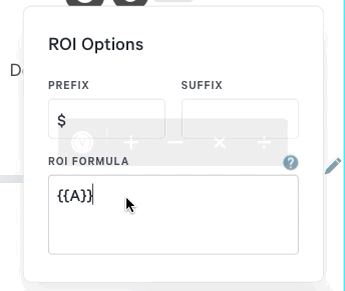### 3. Conversion calculations

This is an optional part of your formula. It will depend on the variables you're using.

Each of your variables will have a unit of measurement. Here are just a few examples:

• Per day
• Per month
• Per year
• Per hour

When you have variables with different units of measurement, you'll want to include a conversion calculation in your formula. Essentially, this converts variables to the same unit of measurement so they can calculate accurately.

As an example, let's say you're building a formula to show the number of dollars your client will save per year. You have a variable that's "Leads per month." So you'll need to include a conversion in your formula, from the monthly value to the annual one. You can add a multiplication by 12 for that, since 12 months = 1 year.

Add the multiplication operator and type in "12." It will look something like this.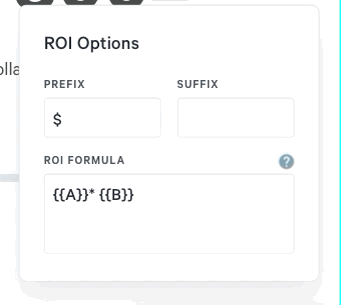Note: You can type in any numbers you need for conversion calculations. You can also manually type in your variables, though using the toolbar helps prevent errors.

As we covered in this guide, you can set each of your variables to represent a range of values. That way you and your client can try out different values within each range.

No matter what range you set, the ROI calculator will use the current value you add for each variable.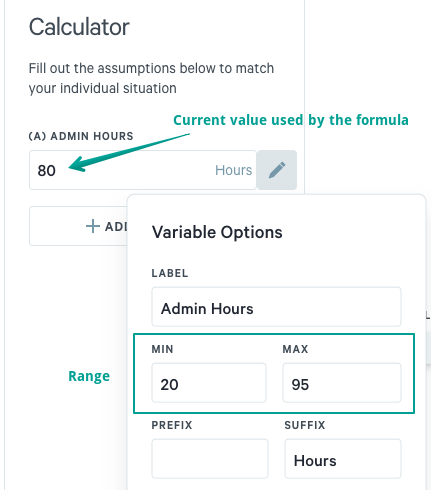You can change the current value or the range at any time.

Note: Once you share the calculator with your client, they'll only be able to change the current value – not the range.

If you've ever used a spreadsheet to make calculations, this process is very similar to that.

Clicking on  Variables brings up a list of your variables. Choose the one you want to add to your formula.

Clicking on any of the calculation operators (+, -, x, or ÷) will add it to your formula.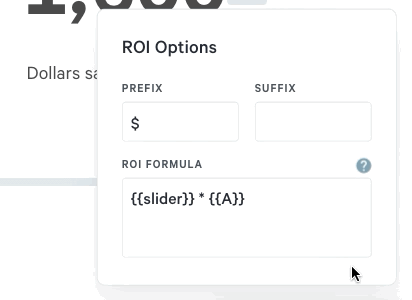You can edit your formula at any time, either using the toolbar or typing manually. You can insert variables and operators anywhere in your formula.

If the current formula can't be calculated with its existing parameters, you'll see an error message.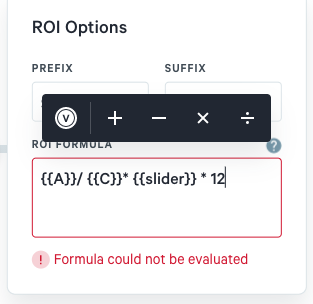This error may appear as you're adjusting your formula. If you make additional changes that re-validate the formula, the error will disappear.

One common source of errors is in the order of calculation. Read on for more details.

## What is calculated first?

The ROI calculator works with your formula in a specific order – it's called the "order of precedence."

1. Anything in parentheses is calculated first
2. Multiplication and division are calculated second
3. Addition and subtraction are calculated third

You can see this in action in the sample formula below.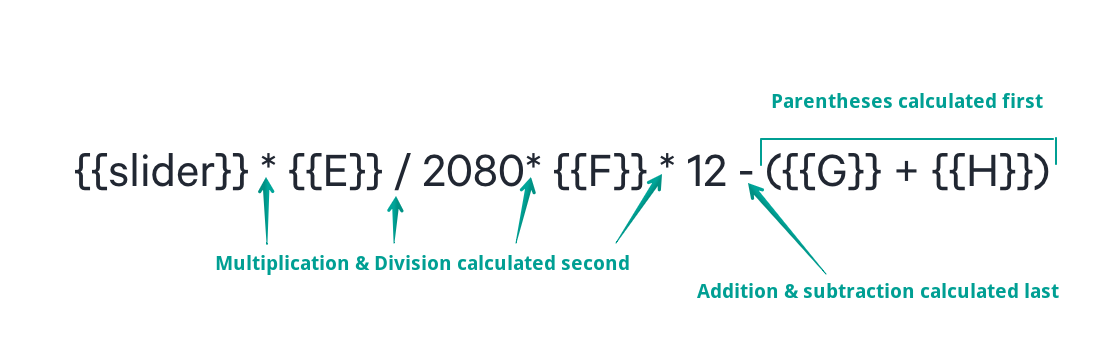As you write your formula, keep this order in mind. If you're seeing a different calculated result than you expect, this order of precedence is often the reason.

Make sure to add parentheses around any parts of your formula that need to be handled first. Then, remember that multiplication/division will always be calculated before addition/subtraction. If you need to have any addition or subtraction happen first, put them in parentheses!

Note: If you do spreadsheet calculations, you might be familiar with the acronym "PEMDAS." It describes the standard order of precedence for spreadsheets. Qwilr's ROI calculator works very similarly, except that it doesn't calculate exponents. So our acronym is more like "PMDAS."

Need more help with formulas? Please reach out to our team.